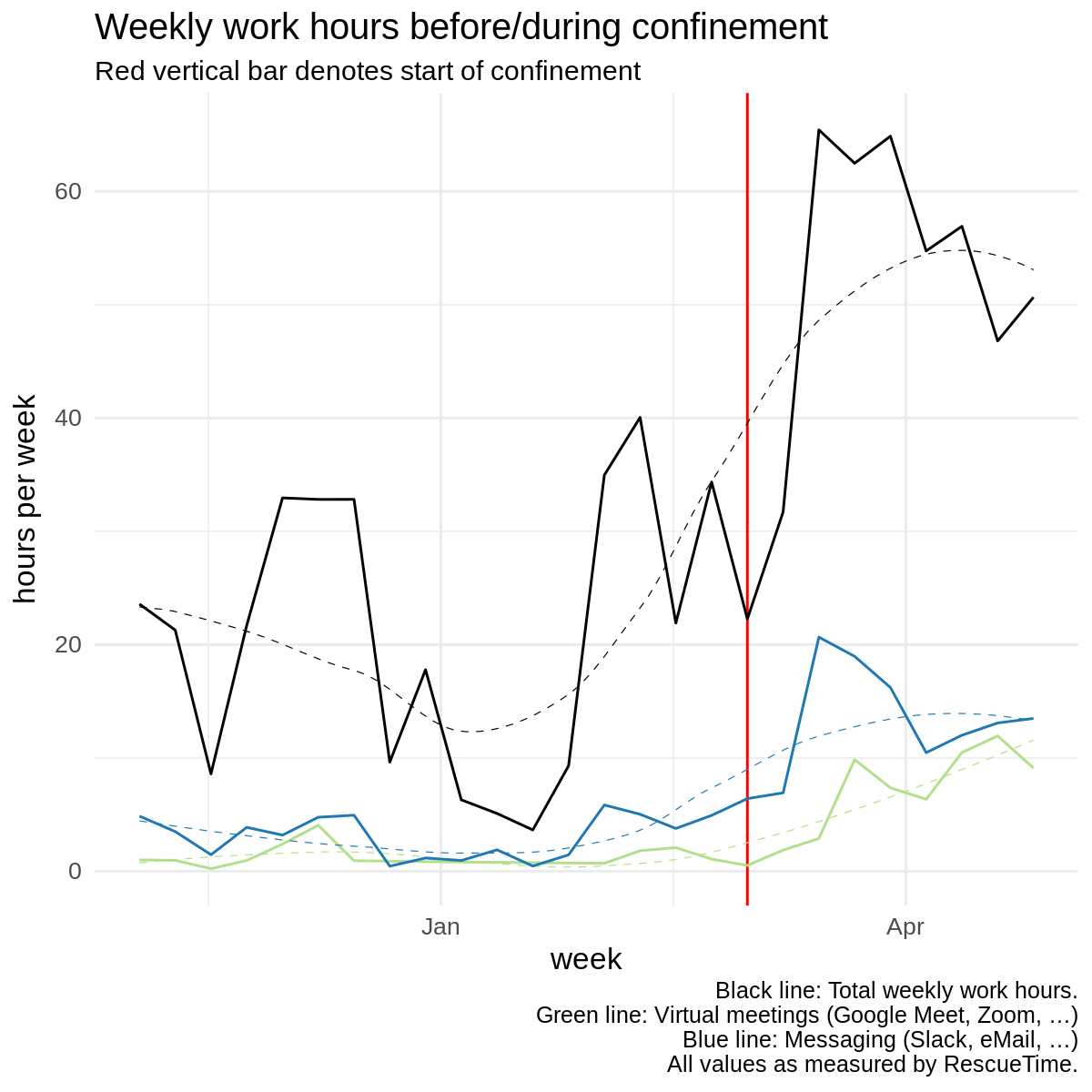# Details for `confinement-measures-rescuetime.ipynb`

#### Description

How do the lockdowns/confinements related to COVID-19 influence our work patterns. This notebook looks into it!

#### Tags & Data Sources

productivity RescueTime connection

# Confinement effects as measured by RescueTime¶

RescueTime is a tool that runs on your computer and tracks which software you're using. Let's see how those values change over time, comparing before and during the confinement. You can easily run this notebook on your data. You just need to connect your RescueTime account to Open Humans!

Below's the boring processing code.

## Prepare RescueTime data¶

Here we're processing the data into a format we can easily plot

```/opt/conda/lib/python3.6/site-packages/rpy2/rinterface/__init__.py:186: RRuntimeWarning:
Attaching package: ‘lubridate’

warnings.warn(x, RRuntimeWarning)
/opt/conda/lib/python3.6/site-packages/rpy2/rinterface/__init__.py:186: RRuntimeWarning: The following object is masked from ‘package:base’:

date

warnings.warn(x, RRuntimeWarning)
```
```/opt/conda/lib/python3.6/site-packages/rpy2/rinterface/__init__.py:186: RRuntimeWarning: Need help getting started? Try the cookbook for R:
http://www.cookbook-r.com/Graphs/

warnings.warn(x, RRuntimeWarning)
/opt/conda/lib/python3.6/site-packages/rpy2/rinterface/__init__.py:186: RRuntimeWarning: `geom_smooth()` using method = 'loess'

warnings.warn(x, RRuntimeWarning)
```# Confinement effects as measured by RescueTime¶

RescueTime is a tool that runs on your computer and tracks which software you're using. Let's see how those values change over time, comparing before and during the confinement. You can easily run this notebook on your data. You just need to connect your RescueTime account to Open Humans!

Below's the boring processing code.

In :
```from ohapi import api
import os
import requests
import tempfile
import json

user_details = api.exchange_oauth2_member(os.environ.get('OH_ACCESS_TOKEN'))
for i in user_details['data']:
if i['source'] == "direct-sharing-149":
```

## Prepare RescueTime data¶

Here we're processing the data into a format we can easily plot

In :
```from datetime import datetime
import pandas as pd
date = []
time_spent_seconds = []
activity = []
category = []
productivity = []
for element in rescuetime_data['rows']:
date.append(element)
time_spent_seconds.append(element)
activity.append(element)
category.append(element)
productivity.append(element)
date = [datetime.strptime(dt,"%Y-%m-%dT%H:%M:%S") for dt in date]

rt_df = pd.DataFrame(data={
'date': date,
'time_spent_seconds': time_spent_seconds,
'activity': activity,
'category': category,
'productivity': productivity
})
```
In :
```%load_ext rpy2.ipython
```
In :
```%%R -i rt_df
library(lubridate)
rt_df\$date <- as.Date(rt_df\$date)
rt_df\$week <- floor_date(rt_df\$date,unit='week')
rt_df <- subset(rt_df, rt_df\$week < as.Date('2020-05-03'))
rt_df <- subset(rt_df, rt_df\$week > as.Date('2019-11-01'))
rt_df_agg <- aggregate(time_spent_seconds~week+activity,data=rt_df,FUN=sum)

rt_df_agg_all <- aggregate(time_spent_seconds~week,data=rt_df,FUN=sum)

meeting_subset_df <- subset(rt_df, rt_df\$activity %in% meeting_activities)
rt_df_agg_meetings <- aggregate(time_spent_seconds~week,data=meeting_subset_df, FUN=sum)

message_activities = c('Slack', 'Mail','rocket.chat')
message_subset_df <- subset(rt_df, rt_df\$activity %in% message_activities)
rt_df_agg_message <- aggregate(time_spent_seconds~week,data=message_subset_df, FUN=sum)
```
```/opt/conda/lib/python3.6/site-packages/rpy2/rinterface/__init__.py:186: RRuntimeWarning:
Attaching package: ‘lubridate’

warnings.warn(x, RRuntimeWarning)
/opt/conda/lib/python3.6/site-packages/rpy2/rinterface/__init__.py:186: RRuntimeWarning: The following object is masked from ‘package:base’:

date

warnings.warn(x, RRuntimeWarning)
```
In :
```%%R -w 6 -h 6 --units in -r 200
library(ggplot2)

ggplot(rt_df_agg_all,aes(x=week,y=time_spent_seconds/60/60)) +
geom_vline(xintercept=as.Date('2020-03-01'), color='red') +
geom_line() +
geom_smooth(se=FALSE,color='black',linetype = "dashed",size=0.2) +
geom_line(data=rt_df_agg_meetings,color='#b2df8a') +
geom_smooth(data=rt_df_agg_meetings,se=FALSE,color='#b2df8a',linetype = "dashed",size=0.2) +
geom_line(data=rt_df_agg_message,color='#1f78b4') +
geom_smooth(data=rt_df_agg_message,se=FALSE,color='#1f78b4',linetype = "dashed",size=0.2) +
theme_minimal() +
scale_y_continuous('hours per week') +
labs(
title = "Weekly work hours before/during confinement",
subtitle = "Red vertical bar denotes start of confinement",
caption = 'Black line: Total weekly work hours.\nGreen line: Virtual meetings (Google Meet, Zoom, …)\nBlue line: Messaging (Slack, eMail, …)\nAll values as measured by RescueTime.'
) + theme(text = element_text(size=12)) +
theme(plot.caption= element_text(size=9))
```
```/opt/conda/lib/python3.6/site-packages/rpy2/rinterface/__init__.py:186: RRuntimeWarning: Need help getting started? Try the cookbook for R:
http://www.cookbook-r.com/Graphs/

warnings.warn(x, RRuntimeWarning)
/opt/conda/lib/python3.6/site-packages/rpy2/rinterface/__init__.py:186: RRuntimeWarning: `geom_smooth()` using method = 'loess'

warnings.warn(x, RRuntimeWarning)
```